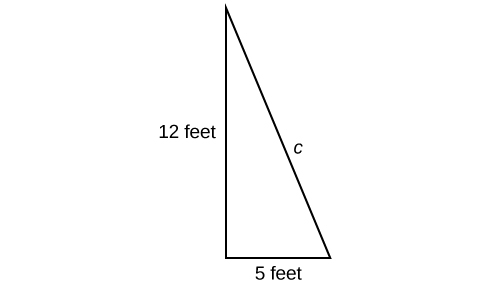## What you’ll learn to do: Simplify radical expressions and solve equations with rational exponents

A hardware store sells 16-ft ladders and 24-ft ladders. A window is located 12 feet above the ground. A ladder needs to be purchased that will reach the window from a point on the ground 5 feet from the building. To find out the length of ladder needed, we can draw a right triangle as shown in Figure 1, and use the Pythagorean Theorem.Figure 1

\begin{align} {a}^{2}+{b}^{2}& = {c}^{2} \\ {5}^{2}+{12}^{2}& = {c}^{2} \\ 169& = {c}^{2} \end{align}

Now we need to find out the length that, when squared, is 169, to determine which ladder to choose. In other word we need to find a square root. In this section we will investigate methods of finding solutions to problems such as this one.

## Contribute!

Did you have an idea for improving this content? We’d love your input.